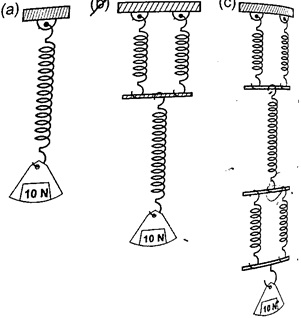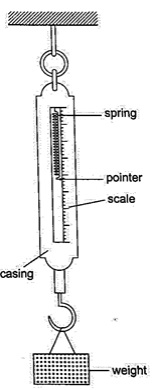# Hooke's law.

### Guest Account

Attempt Form Two Physics Questions
Guest Account

# Hooke's law.

- Hooke’s law states that the extension of a spring is proportional to the applied force, provided that the force is not large enough to deform the spring permanently.
- Mathematically expressed as Force α extension.

### Spring constant

- Since Force α extension then Force / Extension = constant (k).
- The constant of proportionality (k) is called the spring constant.
F / e = k or Force (N) = k e.
- The spring constant is a measure of the stiffness of a spring. The greater the constant the stiffer the spring.
- The spring constant varies with the following;-
1. Material – identical springs mad of different materials will have different constants i.e. steel and copper.
2. Diameter – the stiffness decreases with the increase in diameter.
3. Thickness of the wire – a spring made of a thicker wire is stiffer than the one made of thin wire of the same material.
4. Length of spring – a short spring is stiffer than a longer one.
5. Number of turns per unit length – a spring with higher number of turns per unit length is less stiff than the one with fewer turns per unit length.
Example 1
1. If the springs shown below are similar and the constant of proportionality (k) is 100 Nm-1, determine total extension in each arrangement.Solution
k = 100 Nm-1
extension = force / k = 10 ÷ 100 = 0.1 m = 10 cm. Extension of the lower spring = 10 cm, extension of the two parallel springs = 5 cm.
Total extension = 10 + 5 = 15 cm. Extension of the two lower springs = 5 cm
Middle spring extend by = 10 cm
Upper two springs extend by = 5 cm
Total extension = 5 + 10 + 5 = 20 cm.

The spring balance
- It is made up of a spring mounted in a metal or plastic casing.
- The spring is fitted with a pointer which moves along a calibrated scale divided into ten equal parts.Example 1
1. A load of 4 N causes a certain copper wire to extend by 1.0 mm. Find the load that will cause a 3.2 mm extension on the same wire. (Assume Hooke’s law is obeyed).
Solution
F α e also F1 / F2 = e1 / e2 = F2 = (4 × 3.2) / 1.0 = 12.8 N.

Example 2
2. A body of 200 g was hung from the lower end of a spring which obeys Hooke’s law. Given that the spring extended by 100 mm, what is the spring constant for this spring?
Solution
F = α e, F = k e. F = 200 x 10-3
kg x 10 N /kg = 2 N.
Extension = 100 x 10-3 m = 0.1 m.
Spring constant (k) = 2 / 0.1 = 20 N/m.

Example 3
3. Two identical springs, whose spring constant is 6.0 N/cm, are used to support a load of 60 N as shown below. Determine the extension of each spring.Solution
Since the springs are parallel their spring constant equals 2k.
Therefore extension = Force / k = 2 F / k = 60 / 2 x 6 = 5 cm.
Each spring will extend by 5 cm.